# Ornstein-Zernike relation

Notation used:

•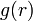$g(r)$ is the pair distribution function.
•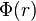$\Phi(r)$ is the pair potential acting between pairs.
•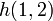$h(1,2)$ is the total correlation function.
•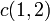$c(1,2)$ is the direct correlation function.
•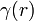$\gamma (r)$ is the indirect (or series or chain) correlation function.
•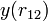$y(r_{12})$ is the cavity correlation function.
•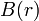$B(r)$ is the bridge function.
•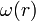$\omega(r)$ is the thermal potential.
•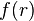$f(r)$ is the Mayer f-function.

The Ornstein-Zernike relation integral equation  is given by: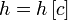$h=h\left[c\right]$

where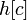$h[c]$ denotes a functional of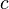$c$. This relation is exact. This is complemented by the closure relation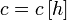$c=c\left[h\right]$

Note that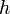$h$ depends on$c$, and$c$ depends on$h$. Because of this$h$ must be determined self-consistently. This need for self-consistency is characteristic of all many-body problems. (Hansen and McDonald, section 5.2 p. 106) For a system in an external field, the Ornstein-Zernike relation has the form (5.2.7)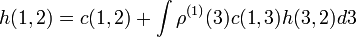$h(1,2) = c(1,2) + \int \rho^{(1)}(3) c(1,3)h(3,2) d3$

If the system is both homogeneous and isotropic, the Ornstein-Zernike relation becomes (Eq. 6 of Ref. 1)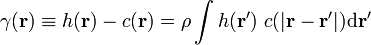$\gamma ({\mathbf r}) \equiv h({\mathbf r}) - c({\mathbf r}) = \rho \int h({\mathbf r'})~c(|{\mathbf r} - {\mathbf r'}|) {\rm d}{\mathbf r'}$

In words, this equation (Hansen and McDonald, section 5.2 p. 107)

"...describes the fact that the total correlation between particles 1 and 2, represented by$h(1,2)$, is due in part to the direct correlation between 1 and 2, represented by$c(1,2)$, but also to the indirect correlation,$\gamma (r)$, propagated via increasingly large numbers of intermediate particles."

Notice that this equation is basically a convolution, i.e.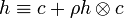$h \equiv c + \rho h\otimes c$

(Note: the convolution operation written here as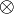$\otimes$ is more frequently written as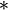$*$) This can be seen by expanding the integral in terms of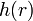$h(r)$ (here truncated at the fourth iteration):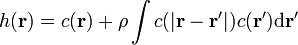$h({\mathbf r}) = c({\mathbf r}) + \rho \int c(|{\mathbf r} - {\mathbf r'}|) c({\mathbf r'}) {\rm d}{\mathbf r'}$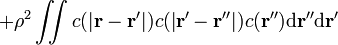$+ \rho^2 \iint c(|{\mathbf r} - {\mathbf r'}|) c(|{\mathbf r'} - {\mathbf r''}|) c({\mathbf r''}) {\rm d}{\mathbf r''}{\rm d}{\mathbf r'}$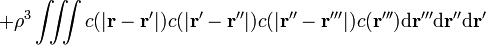$+ \rho^3 \iiint c(|{\mathbf r} - {\mathbf r'}|) c(|{\mathbf r'} - {\mathbf r''}|) c(|{\mathbf r''} - {\mathbf r'''}|) c({\mathbf r'''}) {\rm d}{\mathbf r'''}{\rm d}{\mathbf r''}{\rm d}{\mathbf r'}$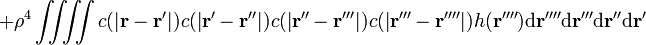$+ \rho^4 \iiiint c(|{\mathbf r} - {\mathbf r'}|) c(|{\mathbf r'} - {\mathbf r''}|) c(|{\mathbf r''} - {\mathbf r'''}|) c(|{\mathbf r'''} - {\mathbf r''''}|) h({\mathbf r''''}) {\rm d}{\mathbf r''''} {\rm d}{\mathbf r'''}{\rm d}{\mathbf r''}{\rm d}{\mathbf r'}$
etc.

Diagrammatically this expression can be written as :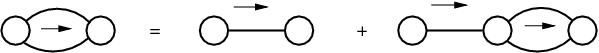where the bold lines connecting root points denote$c$ functions, the blobs denote$h$ functions. An arrow pointing from left to right indicates an uphill path from one root point to another. An `uphill path' is a sequence of Mayer bonds passing through increasing particle labels. The Ornstein-Zernike relation can be derived by performing a functional differentiation of the grand canonical distribution function.

## Ornstein-Zernike relation in Fourier space

The Ornstein-Zernike equation may be written in Fourier space as ( Eq. 5):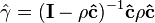$\hat{\gamma} = (\mathbf{I} - \rho \mathbf{\hat{c}})^{-1} \mathbf{\hat{c}} \rho \mathbf{\hat{c}}$

The carets denote the three-dimensional Fourier transformed quantities which reduce explicitly to: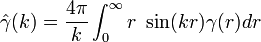$\hat{\gamma} (k) = \frac{4 \pi}{k} \int_0^\infty r~\sin (kr) \gamma(r) dr$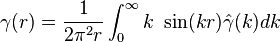$\gamma (r) = \frac{1}{2 \pi^2 r} \int_0^\infty k~\sin (kr) \hat{\gamma}(k) dk$

Note: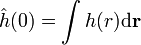$\hat{h}(0) = \int h(r) {\rm d}{\mathbf r}$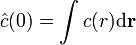$\hat{c}(0) = \int c(r) {\rm d}{\mathbf r}$